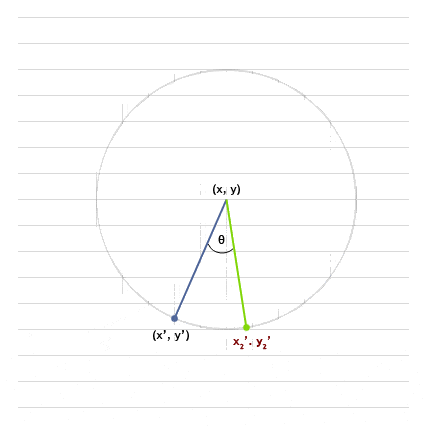# A vector question!

MatrixGLIt's been a while since I've been in a math class. I'm just trying to make a simple openGL demo, and I ran into this problem.

If you look at the picture, I know the values of:

x, y
x', y'
θ

Now, from those, how can I find X2' and Y2' on a cartesian coordinate system?

lol, the question might seem dumb, but I've been out of touch for a long time and while I've done some searches I did not find anything specific.

Any help would be greatly appreciated!

Thanks!

neutrino
Use polar coordinates.

You can find r and angle (of x' and y'), say phi, with (x,y) and (x',y'). r is going to be the same for (x2', y2') and you know the angle, which is phi+theta. So use r and theta+phi to determine x2' and y2'.

Homework Helper
Gold Member
Dearly Missed
If you don't want to use polar coordinates, you might do it this way:
You need 2 equations to determine (x2,y2), so what could work?

1. Both (x',y') and (x2,y2) lie on the SAME circle.
What equation can represent this fact?

2. We may determine the angle at (x',y'):
Having a triangle with two equal sides and a given angle should give us this angle.

3. Set up a dot product between two suitable vectors!
This gives you a second equation, along with the circle equation.